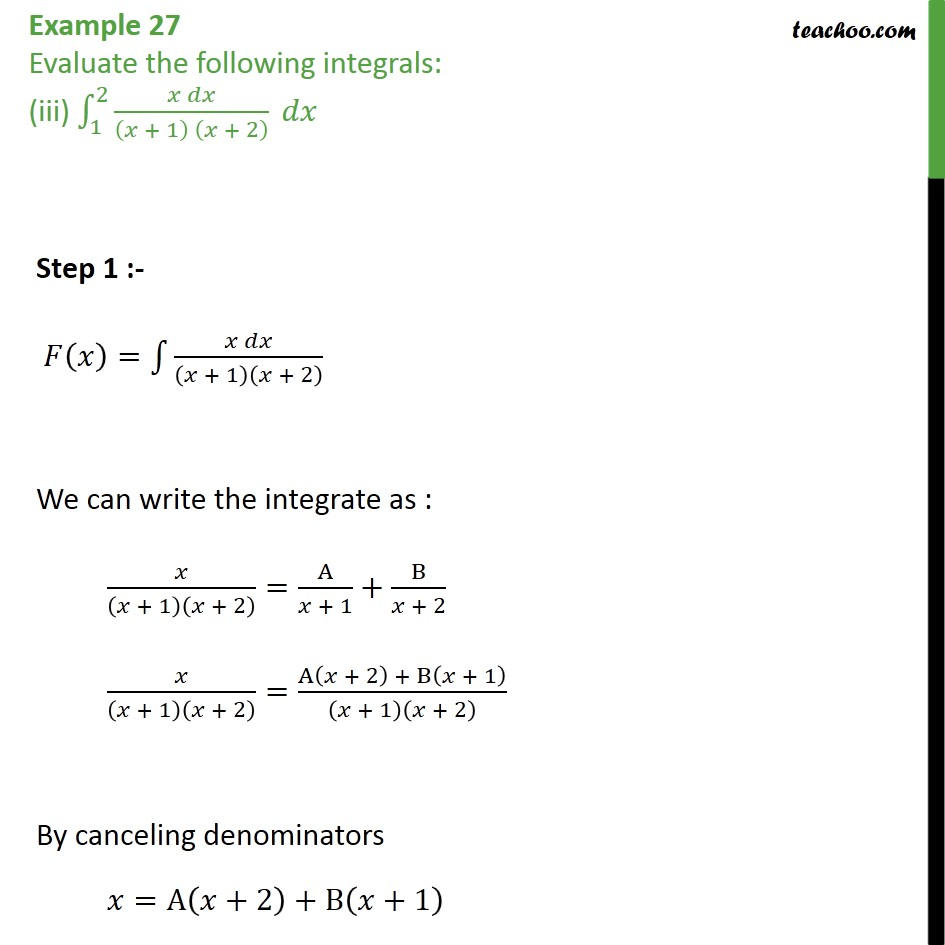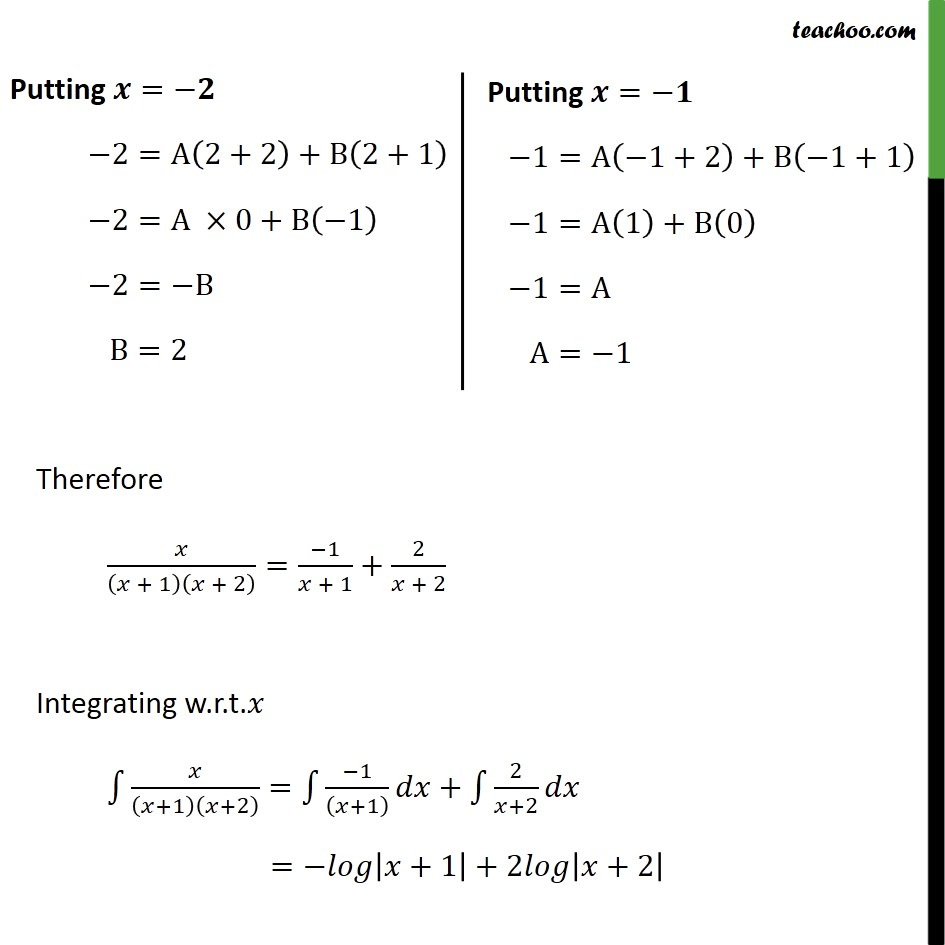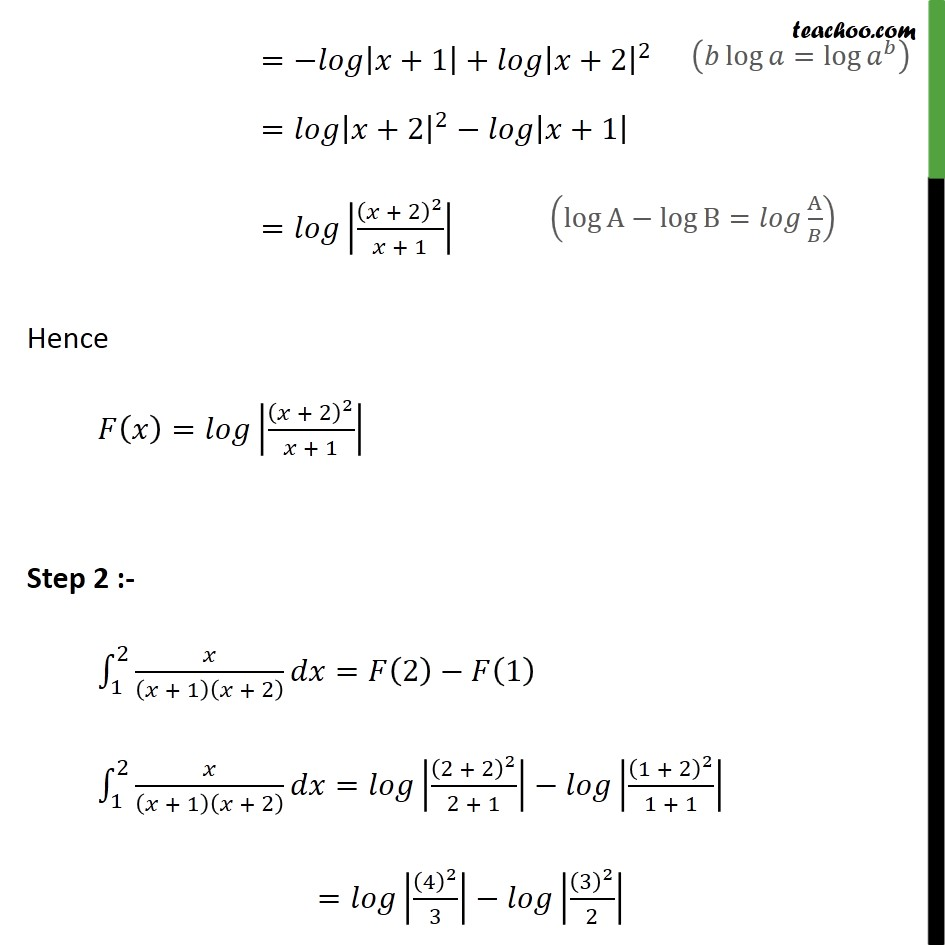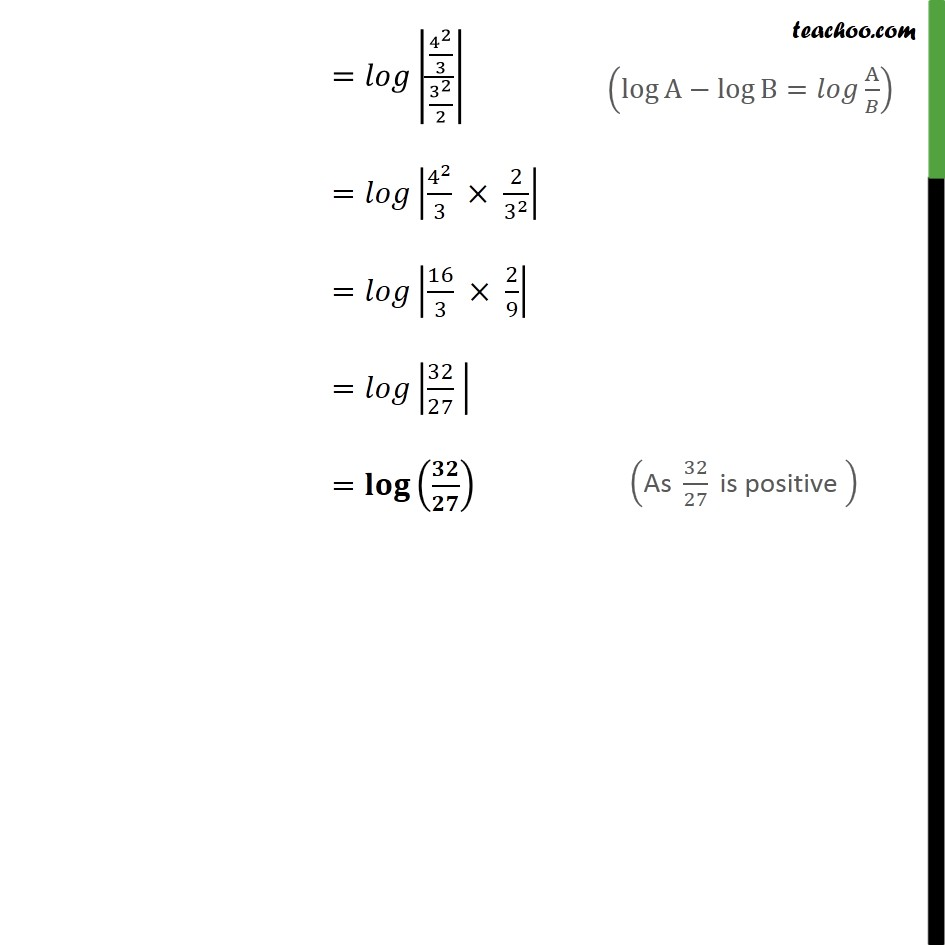1. Chapter 7 Class 12 Integrals
2. Concept wise
3. Definate Integration - By Partial Fraction

Transcript

Example 27 Evaluate the following integrals: (iii) 1 2 + 1 + 2 Step 1 :- = + 1 + 2 We can write the integrate as : + 1 + 2 = A + 1 + B + 2 + 1 + 2 = A + 2 + B + 1 + 1 + 2 By canceling denominators =A +2 +B +1 Therefore + 1 + 2 = 1 + 1 + 2 + 2 Integrating w.r.t. +1 +2 = 1 +1 + 2 +2 = +1 +2 +2 = +1 + +2 2 = +2 2 +1 = + 2 2 + 1 Hence = + 2 2 + 1 Step 2 :- 1 2 + 1 + 2 = 2 1 1 2 + 1 + 2 = 2 + 2 2 2 + 1 1 + 2 2 1 + 1 = 4 2 3 3 2 2 = 4 2 3 3 2 2 = 4 2 3 2 3 2 = 16 3 2 9 = 32 27 =

Definate Integration - By Partial Fraction

About the AuthorDavneet Singh
Davneet Singh is a graduate from Indian Institute of Technology, Kanpur. He has been teaching from the past 9 years. He provides courses for Maths and Science at Teachoo.